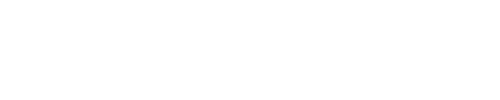# Logistic Regression with Python and Numpy

4.5
stars
128 ratings
Offered ByIn this Guided Project, you will:

Implement Logistic Regression using Python and Numpy.

Apply Logistic Regression to solve binary classification problems.

2 hours
Intermediate
Split-screen video
English
Desktop only

In this 2-hour long project-based course, you will learn how to implement Logistic Regression using Python and Numpy. Logistic Regression is an important fundamental concept if you want break into Machine Learning and Deep Learning. Even though popular machine learning frameworks have implementations of logistic regression available, it's still a great idea to learn to implement it on your own to understand the mechanics of optimization algorithm, and the training and validation process. Since this is a practical, project-based course, you will need to have a theoretical understanding of logistic regression, and gradient descent. We will focus on the practical aspect of implementing logistic regression with gradient descent, but not on the theoretical aspect. By the end of this course, you would create and train a logistic model that will be able to predict if a given image is of hand-written digit zero or of hand-written digit one. The model will be able to distinguish between images or 0s and 1s, and it will do that with a very high accuracy. Not only that, your implementation of the logistic model will also be able to solve any generic binary classiﬁcation problem. You will still have to train model instances on speciﬁc datasets of course, but you won’t have to change the implementation and it will be re-usable. The dataset for images of hand written digits comes from the popular MNIST dataset. This data set consists of images for the 10 hand-written digits (from 0 to 9), but since we are implementing logistic regression, and are looking to solve binary classiﬁcation problems - we will work with examples of hand written zeros, and hand written ones and we will ignore examples of rest of the digits. Note: This course works best for learners who are based in the North America region. We’re currently working on providing the same experience in other regions.

### Skills you will develop

Deep LearningMachine LearningLogistic RegressionPython ProgrammingNumpy

## Learn step-by-step

In a video that plays in a split-screen with your work area, your instructor will walk you through these steps:

1. Introduction

2. Hyperparameters

3. Dataset

4. A Mini Batch of Examples

5. Create Model

6. Forward Pass

7. Backward Pass

8. Update Parameters

9. Check Model Performance

10. Training Loop

## How Guided Projects work

In a split-screen video, your instructor guides you step-by-step